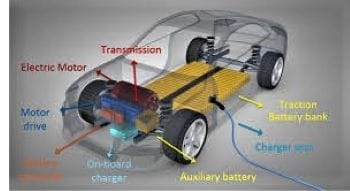# Electric Car Work Principles and Parameters

Here I am looking at the principles of the work of an electric car based on the electric power flow.

Because in another article I have written about the main parts of electric vehicles.  Also, are some key parameters that have to remember here to understand electric cars.

## How Does Electric Car Work

We will look at the bellow figure and will analyze it step by step.The power comes from the AC grid to charge the battery. The flow continues through the AC/DC on-board charger (see electric car components) and DC/DC converter. When the car is in driving mode, the power from the battery goes from the battery DC/DC converter to the high voltage DC bus. Then DC/AC inverter of the motor drive sends the power to the motor.

The motor then converts the electrical energy to the mechanical energy that is sent to the wheels via the transmissions. On the route intervenes a DC/DC uni-directional converter that down on the voltage from the high voltage DC bus to charge the auxiliary battery that aliments the vehicle accessories.

### Electric Car Parameters

#### Battery Capacity

The nominal battery capacity Enom measured in Wh or Kwh , is the total electric energy that can be stored in the battery. Is the maximum filling capacity with the energy of a battery. The battery has a capacity between 10-100 Kwh. The higher the capacity, the more energy the battery can store on the unit of time.
Popular, the name of battery capacity is the energy of the battery.

#### State of charge

The battery state of charge(SoC) is the ratio between the amount of energy currently from the battery, Ebatt, and the battery capacity(total energy), Enom
Bsoc=Ebatt/Enomx100

#### Range

Rmax(Km) is the maximum distance that an electric car can drive when the battery is full. Usually, an electric car work is testing by a standard driving cycle to estimate the range. The range’s unit of measure is miles, kilometers, or other units.

#### Available range

R(Km) is the maximum distance witch a car is unning with the current state of charge of the battery.

#### Energy Consumption Per Kilometer

D(Kwh/Km). When is testing a vehicle by a standardized driven cycle the electric vehicle efficiency is the consumption of energy from the batteries per unit of distance drive.  It could be also the energy from the grid to charge the battery.
The unit of measure is Kwh/Km or Kilowatt-hour per mile.

#### MPGE and Motor Power

MPGE(mile per gallon equivalent) is the distance in miles walking per unit of energy use by vehicles. The rating is based on EPA formula.

Motor Power is the power from the motor to the wheels for propulsion. The power is sensitive or negative depending on the car is driving or under regenerative braking.

Motor power is measured in W(Watt), Kilowatt(Kw), or horsepower.
The motor power is calculated as the product of motor torque, Tm, and motor rotation wm
P=Tmxwm
Tm -measured in Newton-meter(Nm)

Ideally, an electric car must have a high range, low energy consumption per kilometer, and high MPGe.
R=Ebatt/D=Bsoc/100xEnom/D

The battery is the most important part of the electric car. The cost of an electric vehicle is according to it. Understanding the battery performance is very important.

#### The performance parameters that are specifically for the battery:

Nominal Voltage- Vnom(unit of measure is volt V)- It is the voltage rate of the battery when it is full of current. When a battery is discharging or is loading, the voltage reduces gradually to a lower value.

Nominal Current – Inom ( measured in amper A)- It is the rated current of the battery for charging or discharging.

Ampere-Hour- Qnom(measured in Ah)- An ampere-hour is a unit of electric charge that corresponds to the charge transferred by a steady current of one ampere flowing for one hour or 3600 coulombs. The total amount of energy from the battery is calculating by the product of battery ampere-hours and battery nominal voltage. The effective ampere-hour of a battery is determining by the charging/discharging current as is describing by Peukert’s law.
Enom=QnomxVnom

Charge/Discharge Efficiency- ( in%) This parameter refers to the fluctuation rate of the battery current. When charging or discharging the battery, the energy is not constant.

Charge/Discharge Rate- (unit of measure C-rate)- This parameter describes the magnitude of current drawn from or input into the battery, in terms of the nominal ampere-hour of the battery.